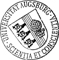## Continuity of f-Projections and Applications to the Iterative Proportional Fitting Procedure

• This paper proves continuity of f-projections and the continuous dependence of the limit matrix of the iterative proportional fitting procedure (IPF procedure) on the given matrix as well as the given marginals under certain regularity constraints. For discrete spaces, the concept of f-projections of finite measures on a compact and convex set is introduced and continuity of f-projections is proven. This result is applied to the IPF procedure. Given a nonnegative matrix as well as row and column marginals the IPF procedure generates a sequence of matrices, called the IPF sequence, by alternately fitting rows and columns to match their respective marginals. If the IPF sequence converges, the application of the previous result yields the continuous dependence of the limit matrix on the given matrix. By generalized convex programming and under some constraints, it is shown that the limit matrix of the IPF sequence continuously depends not only on the given matrix but also on theThis paper proves continuity of f-projections and the continuous dependence of the limit matrix of the iterative proportional fitting procedure (IPF procedure) on the given matrix as well as the given marginals under certain regularity constraints. For discrete spaces, the concept of f-projections of finite measures on a compact and convex set is introduced and continuity of f-projections is proven. This result is applied to the IPF procedure. Given a nonnegative matrix as well as row and column marginals the IPF procedure generates a sequence of matrices, called the IPF sequence, by alternately fitting rows and columns to match their respective marginals. If the IPF sequence converges, the application of the previous result yields the continuous dependence of the limit matrix on the given matrix. By generalized convex programming and under some constraints, it is shown that the limit matrix of the IPF sequence continuously depends not only on the given matrix but also on the marginals.Author: Christoph Gietl, Fabian P. Reffel urn:nbn:de:bvb:384-opus4-23654 https://opus.bibliothek.uni-augsburg.de/opus4/2365 Preprints des Instituts für Mathematik der Universität Augsburg (2013-13) Preprint English Universität Augsburg 2013/06/10 continuity of f-projections; iterative proportional fitting; f-divergence; I-divergence; dependence on input matrix and marginals; limit matrix; I-projection; f-projection Informationstheorie; Kontingenztafelanalyse; Stetigkeit; Statistik Mathematisch-Naturwissenschaftlich-Technische Fakultät Mathematisch-Naturwissenschaftlich-Technische Fakultät / Institut für Mathematik 5 Naturwissenschaften und Mathematik / 51 Mathematik / 510 MathematikDeutsches Urheberrecht mit Print on Demand